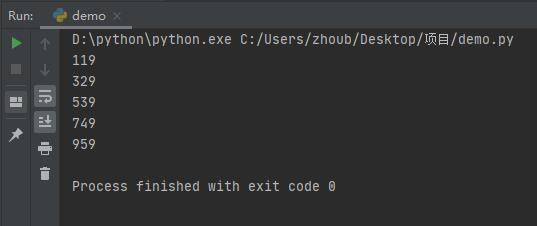python如何求有多少阶梯python求有多少阶梯的方法：

x就是我们想求的阶梯数

for x in range(1,1000):
if (x % 2 == 1) and (x % 3 == 2) and (x % 5 == 4) and (x % 6 == 5) and (x % 7 == 0):
print(x)Python开发开发项目